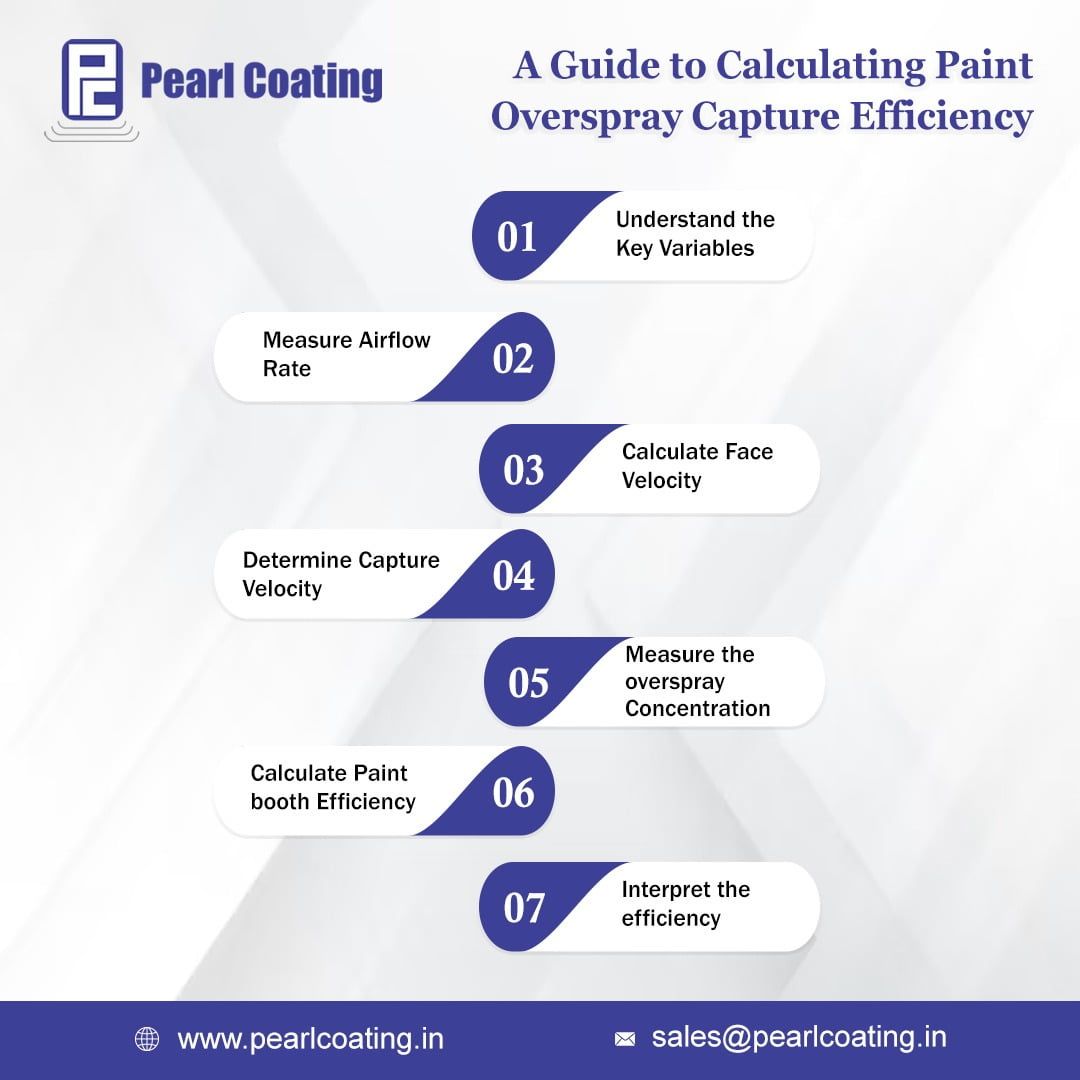## Step-by-Step guide to calculate paint overspray capture efficiency

• Post author:
• Post category:Blog

Calculating paint booth efficiency involves determining how effectively a paint booth system captures overspray and contaminants. Here is a step-by-step guide to help you calculate paint booth efficiency:

Step 1: Understand the Variables Before calculating paint booth efficiency, it’s important to understand the variables involved. The key variables are:

• Airflow rate (Q): The volume of air passing through the booth in a given time, usually measured in cubic feet per minute (CFM).
• Face velocity (Vf): The speed of the air entering the booth, typically measured in feet per minute (FPM).
• Capture velocity (Vc): The minimum velocity required to capture and contain overspray and contaminants, also measured in FPM.
• Cross-sectional area (A): The area through which air enters the booth, typically measured in square feet (ft²).
• Overspray concentration: The amount of overspray or contaminants in the booth, often expressed as grams per cubic meter (g/m³).

Step 2: Measure Airflow Rate Measure the airflow rate (Q) using an anemometer or by referring to the specifications of the paint booth system. The airflow rate determines how quickly air is moving through the booth.

Step 3: Calculate Face Velocity Calculate the face velocity (Vf) by dividing the airflow rate (Q) by the cross-sectional area (A) of the booth’s air intake. The formula is:

Vf = Q / A

Step 4: Determine Capture Velocity Determine the capture velocity (Vc) based on the specific requirements of your paint booth system. Capture velocity varies depending on the type of booth and the materials being sprayed. The manufacturer’s guidelines or industry standards can help you determine the appropriate capture velocity.

Step 5: Measure the overspray concentration using appropriate sampling techniques and equipment. This step helps quantify the amount of overspray or contaminants present in the booth.

Step 6: To calculate the paint booth efficiency, compare the capture velocity (Vc) to the face velocity (Vf). If Vf is higher than Vc, the booth may be less efficient in capturing overspray and contaminants. The efficiency (E) can be calculated using the following formula: E = (Vc / Vf) * 100

Step 7: Interpret the Efficiency: The efficiency value obtained in the previous step represents the percentage of overspray and contaminants captured by the booth. A higher efficiency value indicates better containment and reduced pollution.

It’s important to note that paint booth efficiency can be influenced by various factors, such as booth design, filters, exhaust systems, and operator practices. Regular maintenance, filter replacement, and adherence to manufacturer guidelines are essential for maintaining optimal paint booth efficiency.